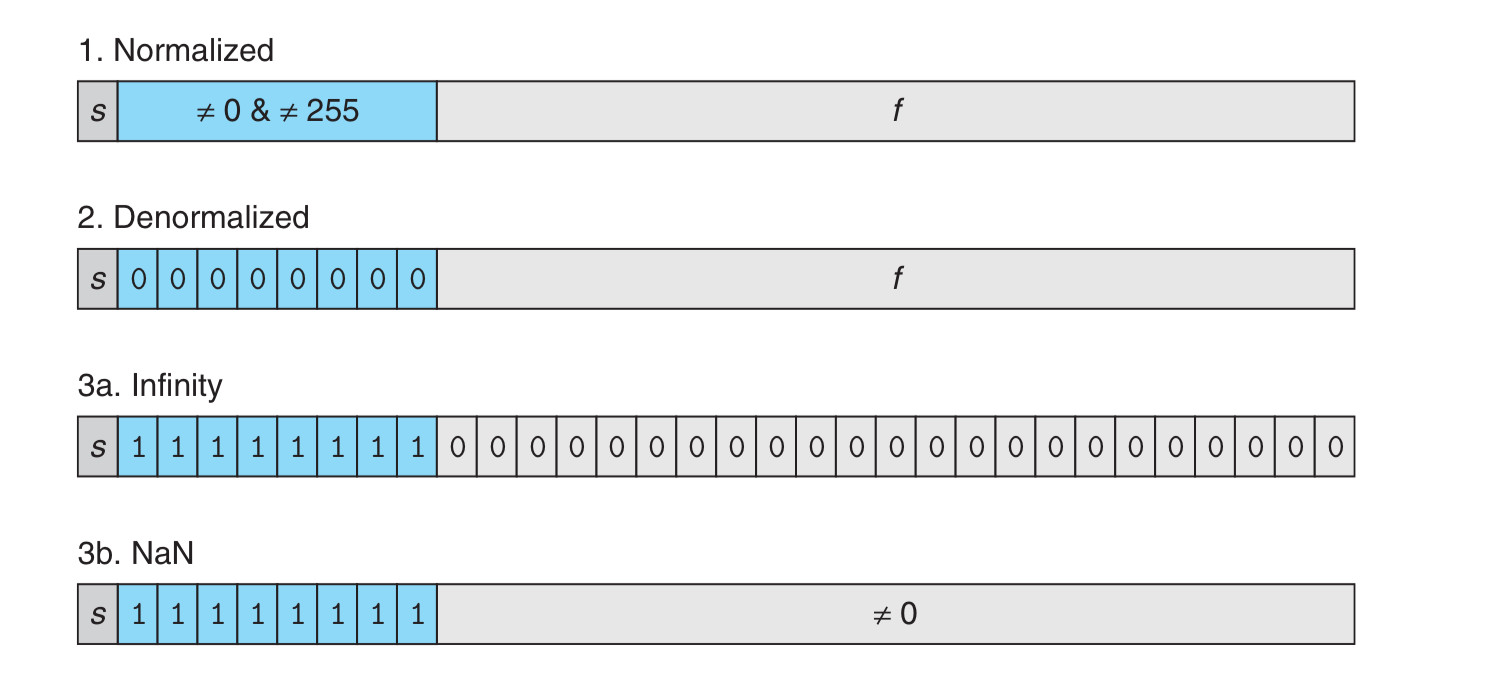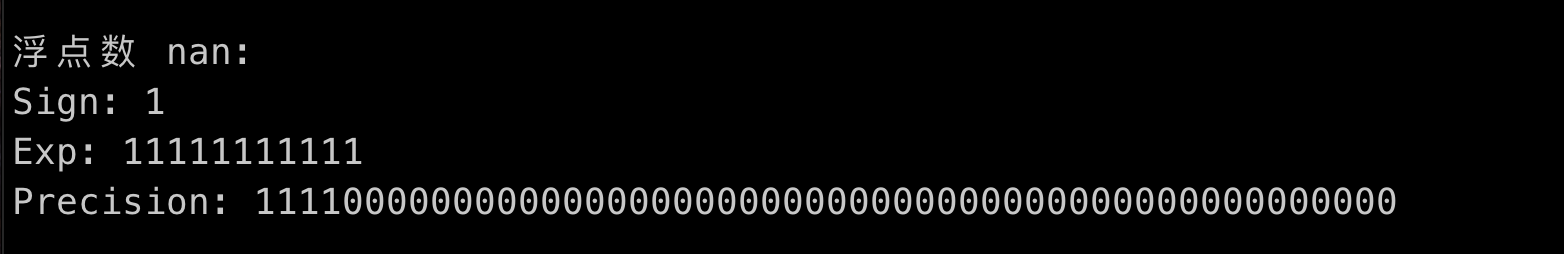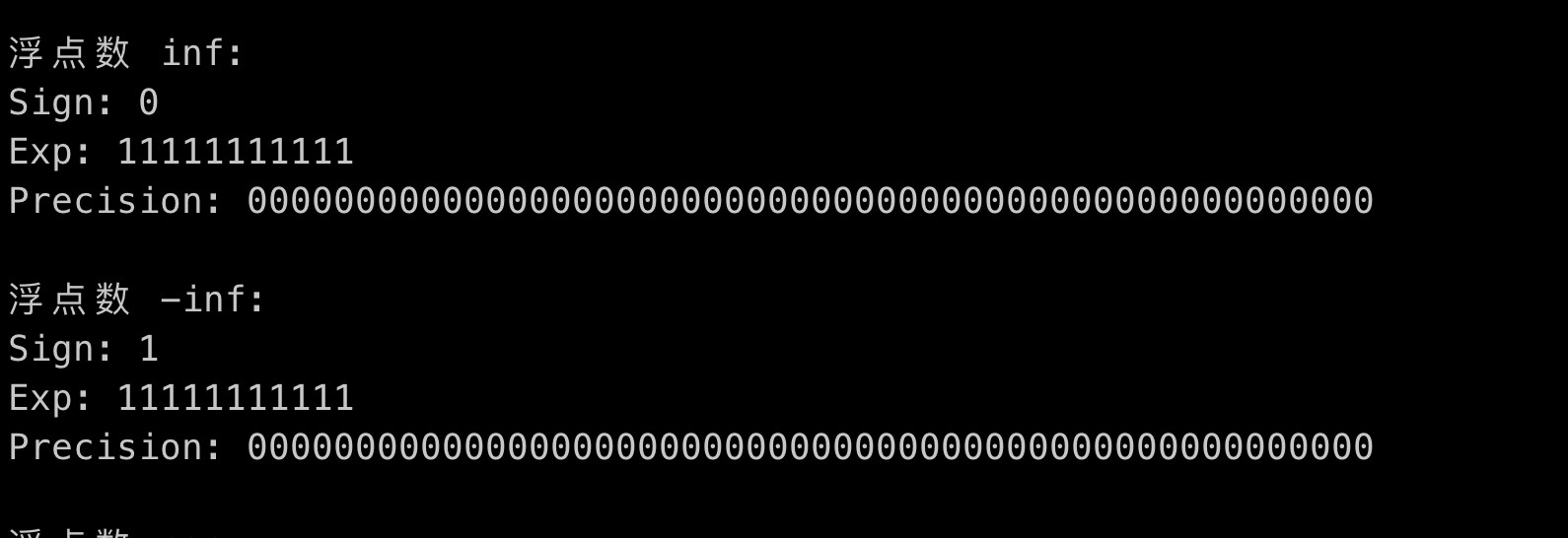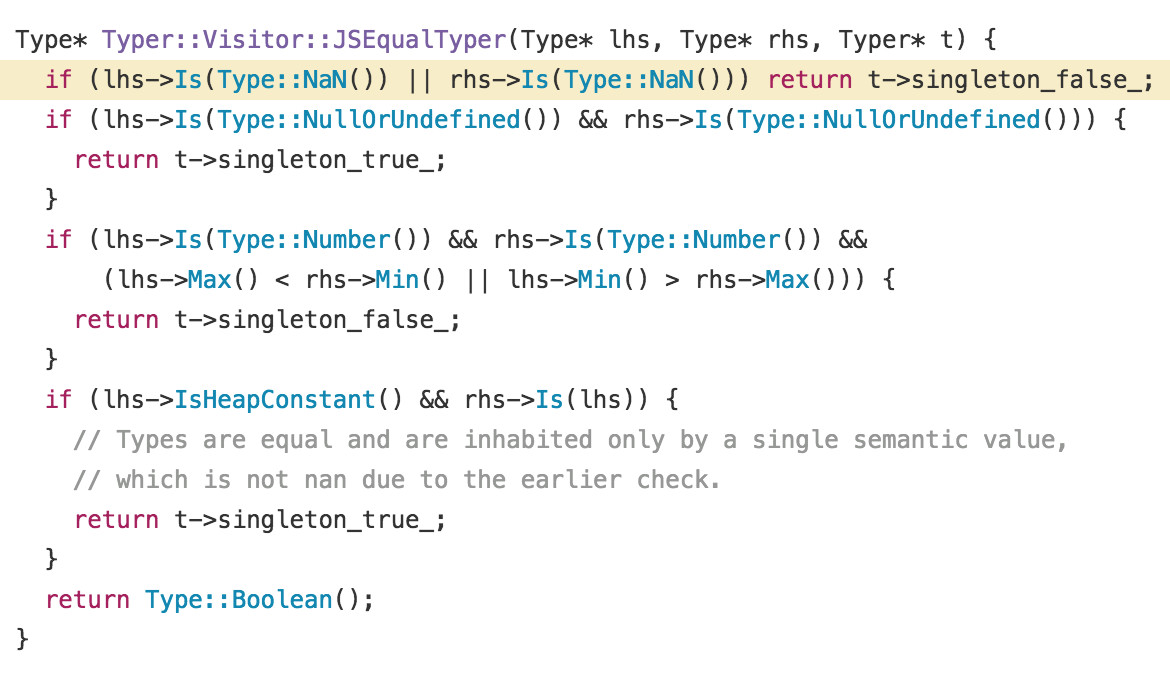# 置顶Under the Hood: NaN of JS精选热门

1、typeof (1 / undefined) 是多少
2、[1,2,NaN].indexOf(NaN) 输出什么

### NaN 的本质1、符号位
2、指数位
3、精度位

``````double createNaN() {
unsigned char *bits = calloc(sizeof(double), 1);
// 大部分人的电脑是小端，所以要从 6 和 7 开始，而不是 0 和 1
// 不清楚概念的可以参考阮老师：
// [理解字节序 - 阮一峰的网络日志](http://www.ruanyifeng.com/blog/2016/11/byte-order.html)
bits = 255;
bits = 255;
unsigned char *start = bits;

double nan = *(double *)(bits);
output(nan);
free(bits);
return nan;
}
``````### 两种 NaN

1、Quiet NaN
2、Signaling NaN

Filling uninitialized memory with signaling NaNs would produce the invalid operation exception if the data is used before it is initialized Using an sNaN as a placeholder for a more complicated object , such as: A representation of a number that has underflowed A representation of a number that has overflowed Number in a higher precision format A complex number

### NaN != NaN

``````_Bool isnan(double whatever) {
long long num = *(long long *)(&whatever); // 浮点数不能进行位运算，所以要改成整数类型，同时保留内部的二进制组成
long long fmask = 0xfffffffffffff; // 不要数了，13 个 f，52 个 1
long long emask = 0x7ff; // 11 个 1
num <<= 1;
num >>= 1; // 清除符号位
}
``````

### 实际情况下的 NaN != NaN 的实现

``````#define isnan(x)    \
(sizeof (x) == sizeof(float)    ?  __inline_isnanf((float)(x))  \
:  sizeof (x) == sizeof(double)  ?      __inline_isnand((double)(x))  \
:   __inline_isnan ((long double)(x)))

static __inline__ int __inline_isnanf( float __x ) {
return __x != __x;
}
static __inline__ int __inline_isnand( double __x ) {
return __x != __x;
}
static __inline__ int __inline_isnan( long double __x ) {
return __x != __x;
}
``````

1、硬件支持 NaN 异常，所以永远都是 false
2、像下文中提到的 V8 的实现方式

``````const obj = { a: 1, b: 2 };
let { c, d } = obj;
c *= 100;
d *= 100;
console.log(c === d);
````````````Number.isNaN = function(value) {
return value !== value;
}
``````